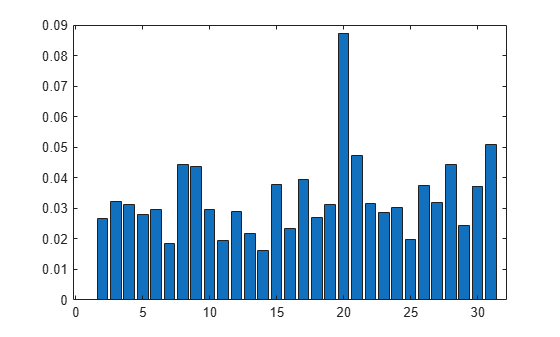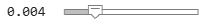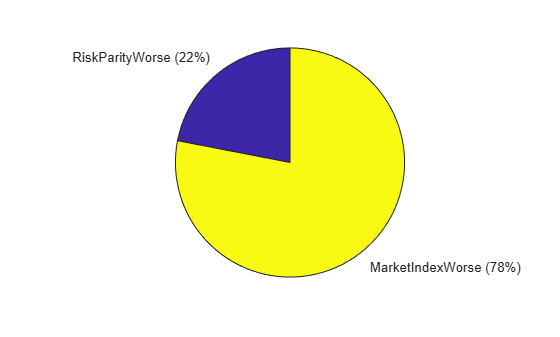# Backtest Using Risk-Based Equity Indexation

This example shows how to use backtesting with a risk parity or equal risk contribution strategy rebalanced approximately every month as a risk-based indexation. In this example, you use the backtesting engine (`backtestEngine`) to create the risk parity strategy, that all assets in the portfolio contribute equally to the risk of the portfolio at each rebalancing period.

To highlight advantages, the example compares the proposed risk-based indexation against a capitalization-weighted indexation. In contrast to a risk parity strategy, the assets of capitalization-weighted portfolios are weighted with respect to the market portfolio. One disadvatage of capitalization-weighted indexation is that it is a trend-following strategy that leads to bubble-risk exposure as the best performers represent a larger proportion of the market. Also, capitalization-weighted indexation can sometimes lead to weights concentratng in a sector. A risk-based indexation is an alternative investment strategy to avoid these issues of capitalization-weighted indexation.

### Define Assets

```% Read the table of daily adjusted close prices for 2006 DJIA stocks. T = readtable('dowPortfolio.xlsx'); % Convert the table to a timetable. pricesTT = table2timetable(T); % Define the number of assets (not counting the market index). nAssets = size(pricesTT,2)-1;```

### Define Risk Parity Strategy

To obtain the long-only fully invested risk parity portfolio, use the `riskBudgetingPortfolio` function. When you pass a returns covariance matrix to `riskBudgetingPortfolio`, the function computes the associated risk parity portfolio. To compute the initial weights for the risk parity strategy, `riskBudgetingPortfolio` uses the covariance matrix estimated with information from the first two months.

```% Set backtesting warm-up period to two 21-day months. warmupPeriod = 21*2; % Set warm-up partition of timetable. % Invest only in assets. First column of pricesTT is the market index. warmupTT = pricesTT(1:warmupPeriod,2:end); % Estimate the covariance matrix. assetReturns = tick2ret(warmupTT); assetCov = cov(assetReturns{:,:}); % Compute the initial risk parity portfolio. initialRiskParity = [0; riskBudgetingPortfolio(assetCov)];```

Visualize initial risk parity portfolio weights.

```% Plot a bar chart. bar(initialRiskParity)```Create the risk parity backtesting strategy using a `backtestStrategy` object. Set the risk parity strategy to rebalance every month.

```% Rebalance frequency rebalFreq = 21; % Every month```

To gather enough data, set the lookback window to at least 2 months. To remove old data from the covariance estimation, set the lookback window to no more than 6 months.

```% Lookback window lookback = [42 126];```

Use a fixed transaction cost equal to 0.5% of the amount traded.

```% Fixed transaction cost transactionCost = 0.005;```

Define the risk parity allocation strategy using the `riskParityFcn` rebalancing function that is defined in Local Functions. Notice that the `riskBudgetingPortfolio` function is at the core of `riskParityFcn.`

```% Risk parity allocation strategy stratRP = backtestStrategy('Risk Parity', @riskParityFcn, ... RebalanceFrequency=rebalFreq, ... LookbackWindow=lookback, ... TransactionCosts=transactionCost, ... InitialWeights=initialRiskParity);```

### Define Benchmark Strategy

Next, compare the risk parity strategy against the portfolio that follows the Dow Jones Industrial Average (DJI) market index. To obtain the market-following portfolio, invest all assets to the first entry of the weights vector because the first column of the prices timetable (`pricesTT`) represents the market index.

```% Compute the initial market portfolio. initialMkt = [1;zeros(nAssets,1)];```

Define the benchmark strategy using the `mktFcn` rebalancing function that is defined in Local Functions.

```% Market index allocation strategy stratBmk = backtestStrategy('Market Index', @mktFcn, ... RebalanceFrequency=rebalFreq, ... LookbackWindow=lookback, ... TransactionCosts=transactionCost, ... InitialWeights=initialMkt);```

### Run Backtest

Run the backtest using a `backtestEngine` object and `runBacktest`.

```% Define strategies for backtest engine. strategies = [stratBmk stratRP]; % Define a backtest engine. backtester = backtestEngine(strategies); % Run the backtest. backtester = runBacktest(backtester,pricesTT,Start=warmupPeriod);```

Use `equityCurve` to plot the equity curve.

```% Plot equity curve. equityCurve(backtester)```The risk parity strategy outperforms the market index by the end of the investment period. To understand why, you can look at which strategy performs better in the event of meaningful losses.

```% Get returns timetable. returnsTT = backtester.Returns; % Determine levels of losses to consider. cutoff =0.004; % Obtain scenarios with losses below cutoff. idxLoss = returnsTT.Market_Index <= -cutoff | ... returnsTT.Risk_Parity <= -cutoff; % Indices when risk parity outperforms the market idxLossMarketWorse = ... returnsTT.Market_Index(idxLoss) <= returnsTT.Risk_Parity(idxLoss); catLoss = categorical(idxLossMarketWorse, ... [0 1], {'RiskParityWorse','MarketIndexWorse'}); % Plot pie chart. pie(catLoss)```The plot shows that in 78% of the scenarios with meaningful losses, the market index loses more than the risk parity strategy. In this example, the reason the risk parity strategy outperforms the market index is because the risk parity strategy is more resilient to larger losses.

### Local Functions

```function new_weights = riskParityFcn(~,pricesTT) % Risk parity rebalance function % Invest only in assets. First column of pricesTT is the market index. assetReturns = tick2ret(pricesTT(:,2:end)); assetCov = cov(assetReturns{:,:}); % Do not invest in the market index. new_weights = [0; riskBudgetingPortfolio(assetCov)]; end function new_weights = mktFcn(~,pricesTT) % Market index rebalance function % Invest only in the market index. new_weights = zeros(size(pricesTT,2),1); new_weights(1) = 1; end % The API of the rebalance functions (riskParityFcn and mktFcn) require % a first input with the current weights. They are % redundant for these two strategies and can be ignored.```